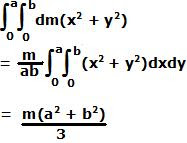## Tuesday, November 11, 2008

### Irodov Problem 1.238Let us consider an infinitesimally small piece of the rod of length dx at a distance x from the axis of rotation. Its mass is given by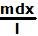as shown in the figure. The moment of inertia of the rod is given by,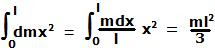(b)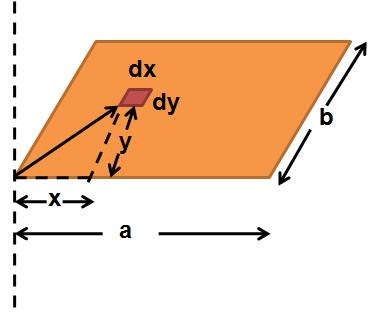Consider an infinitesimally small piece of the plate whose width and length are dx and dy respectively located at coordinates (x,y) if we consider the corner through which the axis passes as the origin as shown in the figure. The distance of this point from the orgin is given by.
The mass of this infinitesimally small piece is given by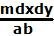. So the moment of inertia of the plate is given by,